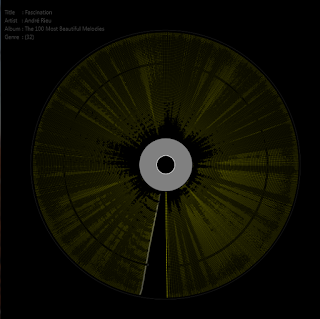### JavaFx Music Spectrum

My first 2018 post, and hi to you all.

Building a circular progress indicator specifically for showing the music progress was the initial idea which  was fairly simple to do with the Animation class ,with the code snippet below

``` Animation = new Transition() {
{
setCycleDuration(Duration.seconds(5));
setInterpolator(Interpolator.LINEAR);
}

@Override
protected void interpolate(double frac) {

double interpolation_angle  = slerpFunc(frac);

double  sinValue = Math.sin(Math.toRadians(interpolation_angle  + startAngle));
double  cosValue = Math.cos(Math.toRadians(interpolation_angle  + startAngle));

double angleX = center2d.getX() + (radius * 0.4) * sinValue;
double angleY = center2d.getY() + (radius * 0.4) * cosValue;

gc.fillOval(angleX,angleY,1,1);
}

};
```

My Slerp function looks like so, found on stackoverflow.

```private double slerpFunc(double frac){

return (((((360 - 0) % 360) + 540 ) % 360) + 180) * frac;
}
```

Half  way the project i decided to change to the project to visualize spectrum magnitude of each band in a song.I used the default number of bands 128 and a threshold of -300 for better visibility.

The bands are drawn from the center creating 128 nodes each with an opacity that corresponds to the spectral threshold.Since the opacity value is between 0 to 1,i had to normalize the threshold values.Andre -Fascination Javafx Music Spectrumsleep away Spectrum